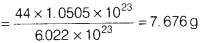# The number of molecules in 0.25 mole of ammonia

Calculate

1. the mass of 1.0505 x 1023 molecules of carbon dioxide (CO2).
[Molar mass of CO2 = 44 g]
2. the number of molecules in 0.25 mole of ammonia (NH3).
3. the formula unit mass of Na2SO3.
[Atomic mass of Na = 23 u, S = 32 u, O = 16 u, H = lu, NA = 6.022 x 1023 mol –1]
1. Mass of 1.0505 x 1023 molecules of CO22. ∵ 1 mole of NH3 have molecule = 6.022 x 1023 x 0.25
= 1.5055 x 1023 molecules
3. Formula unit mass of Na2SO3 = 2 x 23 + 32 + 3 x 16
= 46 + 32 + 48 = 126 amu